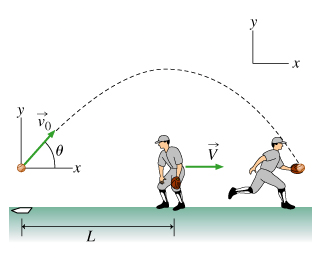# Problem: A softball is hit over a third baseman's head with some speed v0 at an angle θ above the horizontal. Immediately after the ball is hit, the third baseman turns around and begins to run at a constant velocity V = 7.00m/s. He catches the ball t = 2.00s later at the same height at which it left the bat. The third baseman was originally standing L = 18.0m from the location at which the ball was hit.Part A. Find v0. Use g = 9.81 m/s2 for the magnitude of the acceleration due to gravity. Express the initial speed numerically in units of meters per second to three significant figures.Part B. Find the angle θ in degrees. Express your answer numerically in degrees to three significant figures.

###### FREE Expert Solution

Uniform accelerated motion (UAM) equations, a.k.a. "kinematics equations":

Range:

$\overline{){\mathbf{R}}{\mathbf{=}}\frac{{{\mathbf{v}}_{\mathbf{0}}}^{\mathbf{2}}\mathbf{s}\mathbf{i}\mathbf{n}\mathbf{2}\mathbf{\theta }}{\mathbf{g}}}$

Part A.

From the third kinematic equation, a = 0 m/s2 since the velocity is constant for the motion in the first 2s.

Δx = v0t = (7.00)(2.00) = 14.0 m

The total distance traveled by the ball is given by:

d = L + Δx = 18 + 14 = 32.0 m

This distance is covered in 2.00s

Using the third kinematic equation still and knowing that a = 0 m/s2,

v0x = Δx/t = 32.0/2 = 16.0 m/s

97% (107 ratings)###### Problem Details

A softball is hit over a third baseman's head with some speed v0 at an angle θ above the horizontal. Immediately after the ball is hit, the third baseman turns around and begins to run at a constant velocity V = 7.00m/s. He catches the ball t = 2.00s later at the same height at which it left the bat. The third baseman was originally standing L = 18.0m from the location at which the ball was hit.Part A. Find v0. Use g = 9.81 m/s2 for the magnitude of the acceleration due to gravity. Express the initial speed numerically in units of meters per second to three significant figures.

Part B. Find the angle θ in degrees. Express your answer numerically in degrees to three significant figures.